Next: Phase Diagrams Up: Multi-Phase Systems Previous: Equilibrium Between Phases

# Clausius-Clapeyron Equation

It is possible to characterize the phase-equilibrium line in terms of a differential equation. Referring to Figure 9.1, consider a point, such as, that lies on the phase-equilibrium line, and corresponds to the temperatureand the pressure. The condition (9.63) implies that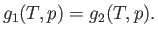(9.64)

Now, consider a neighboring point, such as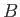, that also lies on the phase-equilibrium line, and corresponds to the temperature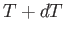and the pressure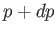. The condition (9.63) yields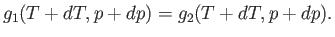(9.65)

Taking the difference between the previous two equations, we obtain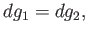(9.66)

where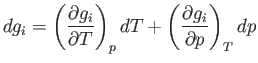(9.67)

is the change in Gibbs free energy per mole of phase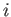in going from pointto point.

The change,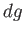, for each phase can also be obtained from the fundamental thermodynamic relation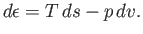(9.68)

Here,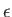refers to molar energy (i.e., energy per mole),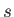to molar entropy, and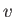to molar volume. Thus,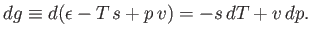(9.69)

Hence, Equation (9.66) implies that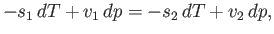(9.70)

or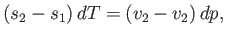(9.71)

which reduces to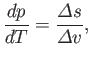(9.72)

where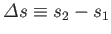and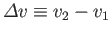. This result is known as the Clausius-Clapeyron equation.

Consider any point on the phase-equilibrium line at temperatureand pressure. The Clausius-Clapeyron equation then relates the local slope of the line to the molar entropy change,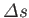, and the molar volume change,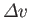, of the substance in crossing the line at this point. Note, incidentally, that the quantities on the right-hand side of the Clausius-Clapeyron equation do not necessarily need to refer to one mole of the substance. In fact, both numerator and denominator can be multiplied by the same number of moles, leaving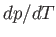unchanged.

Because there is an entropy change associated with a phase transformation, heat must also be absorbed during such a process. The latent heat of transformation,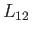, is defined as the heat absorbed when a given amount of phase 1 is transformed to phase 2. Because this process takes place at the constant temperature, the corresponding entropy change is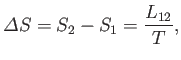(9.73)

whereis the latent heat at this temperature. Thus, the Clausius-Clapeyron equation, (9.72), can also be written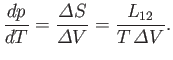(9.74)Next: Phase Diagrams Up: Multi-Phase Systems Previous: Equilibrium Between Phases
Richard Fitzpatrick 2016-01-25